# Display in Jupyter¶

## Plotting in Jupyter¶

#### This magic configures matplotlib to render its figure inline¶

In :
%matplotlib inline

In :
import matplotlib.pyplot as plt
import numpy as np

In :
plt.figure(figsize=(16, 8))

ax0 = plt.subplot2grid((1, 2), (0, 0))
ax1 = plt.subplot2grid((1, 2), (0, 1), polar=True)

x = np.linspace(0, 2 * np.pi, 1000)
y = np.sin(x**2)
ax0.plot(x, y)
plt.polar(x, y, color="r")

plt.show()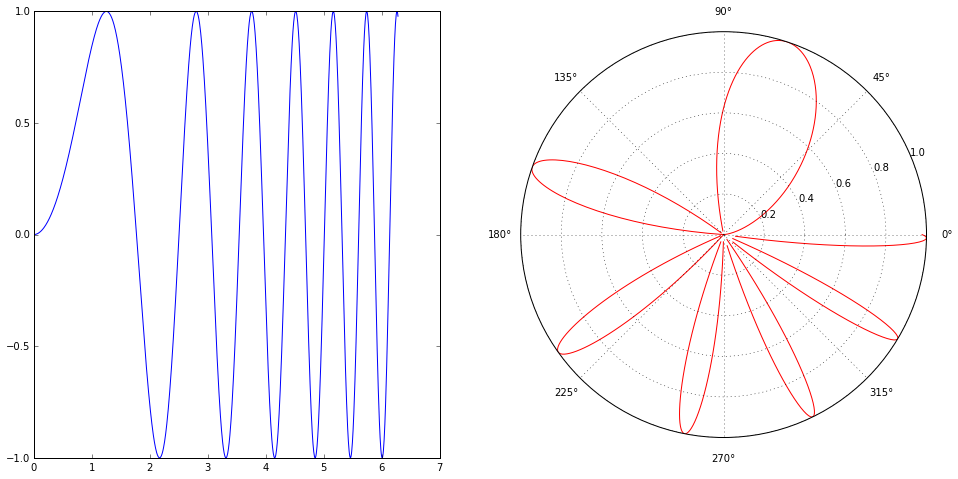## LaTeX equations¶

Courtesy of MathJax, you can include mathematical expressions both inline: $e^{i\pi} + 1 = 0$ and displayed:

$$e^x=\sum_{i=0}^\infty \frac{1}{i!}x^i$$

## General HTML¶

Because Markdown is a superset of HTML you can even add things like HTML tables:

row 1, cell 1 row 1, cell 2
row 2, cell 1 row 2, cell 2

## Local files¶

If you have local files in your Notebook directory, you can refer to these files in Markdown cells via relative URLs.
For example, in the figs subdirectory there is a Python logo:and a video with the HTML5 video tag (WAT: A lightning talk by Gary Bernhardt from CodeMash 2012):

## Basic display imports¶

The display function is tool for displaying images, videos, web pages, etc.

#### Images (JPEG, PNG):¶

In :
from IPython.display import Image

In :
logo = Image(filename='figs/python-logo.png')

In :
logo

Out:In :
Image(url='https://www.python.org/static/community_logos/python-logo-master-v3-TM-flattened.png',
embed=True)

Out:#### Videos¶

In :
from IPython.display import YouTubeVideo

Out:

#### Web pages¶

You can even embed an entire page from another site in an iframe; for example this is the Ipython webpage:

In :
from IPython.display import IFrame
IFrame('http://ipython.org/notebook.html', 800, 400)

Out:

## Interactive Widgets¶

IPython includes an architecture for interactive widgets that tie together Python code running in the kernel and JavaScript/HTML/CSS running in the browser. These widgets enable users to explore their code and data interactively.

How does this work?

• The first argument to interact is a callable/function
• The keyword arguments to interact are "widget abbreviations"
• These "widget abbreviations" are converted to Widget instances
• These Widgets objects are Python objects that are automatically synchronized with JavaScript MVC objects running in the browser.
• interact simply calls its callable each time any widget changes state
In :
from IPython.html.widgets import interact, interactive, fixed
from IPython.html import widgets

:0: FutureWarning: IPython widgets are experimental and may change in the future.

In :
def f(x):
print(x)

In :
res = interact(f, x=True)

True

In :
res = interact(lambda x: print(x**2),
x=10)

100

In :
res = interact(lambda x: print(x**2),
x=(0, 10, 2))

16

In :
res = interact(f, x='Hello World!')

Hello World!

In :
res = interact(f, x=dict(apple='fruit', orange='color', guitar='instrument'))

instrument

In :
@interact(x=True, y=1.0)
def f(x, y):
print(x, y**2 if x else y)

True 1.0

In :
def f(x, y):
print(x + y)

In :
res = interact(f, x=5, y=fixed(20))

25


#### Modeling a Zombie Apocalypse¶

The model has the following constants:

• Natural death (per day): 0.0001%
• Transmission (per day): 0.0095%
• Resurect: 0.0001%
• Destroy: 0.0001%

We will change the following variables:

• initial human population
• initial zombie population
• initial death population
• daily new births
In :
# Zombie apocalypse modeling
from scipy.integrate import odeint

d = 0.0001  # natural death percent (per day)
B = 0.0095  # transmission percent  (per day)
G = 0.0001  # resurect percent (per day)
A = 0.0001  # destroy percent  (per day)
t  = np.linspace(0, 5., 1000)   # time grid

# Solve the system dy/dt = f(y, t)
def f(y, t):
Si = y
Zi = y
Ri = y
P = y
# The model equations (see Munz et al. 2009)
f0 = P - B*Si*Zi - d*Si
f1 = B*Si*Zi + G*Ri - A*Si*Zi
f2 = d*Si + A*Si*Zi - G*Ri
return [f0, f1, f2]

def zombie_apocalypse(initial_human_population,
initial_zombie_population,
initial_death_population,
daily_new_births):
# initial condition vector
y0 = [initial_human_population, initial_zombie_population, initial_death_population, daily_new_births]
soln = odeint(f, y0, t)
S = soln[:, 0]
Z = soln[:, 1]
R = soln[:, 2]

# Plot the apocalypse
plt.figure()
plt.plot(t, S, label='Living')
plt.plot(t, Z, label='Zombies')
plt.xlabel('Days from outbreak')
plt.ylabel('Population')
plt.title('Zombie Apocalypse')
plt.legend(loc=0)

In :
res = interact(zombie_apocalypse,
initial_human_population=widgets.IntSlider(min=500, max=1000, step=50, value=500),
initial_zombie_population=fixed(0),
initial_death_population=widgets.IntSlider(min=0, max=100, step=10, value=0),
daily_new_births=widgets.IntSlider(min=0, max=10, step=1, value=0)
)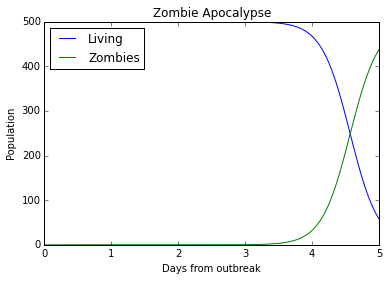## Other ploting libraries¶

### Matplotlib¶

In :
x = np.random.normal(2, 2, 10000)
y = np.random.normal(10, 5, 10000)

In :
def do_histogram(x):
n, bins, patches = plt.hist(x, normed=1)
plt.show()

In :
do_histogram(x)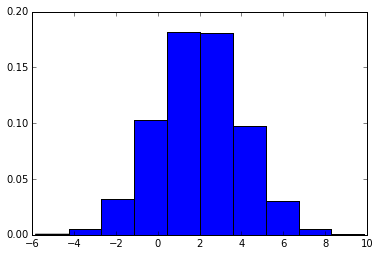### Seaborn¶

In :
import seaborn as sns

In :
do_histogram(x)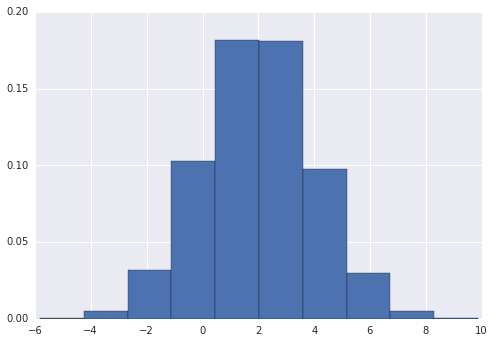In :
p = sns.distplot(x, hist=True, rug=False)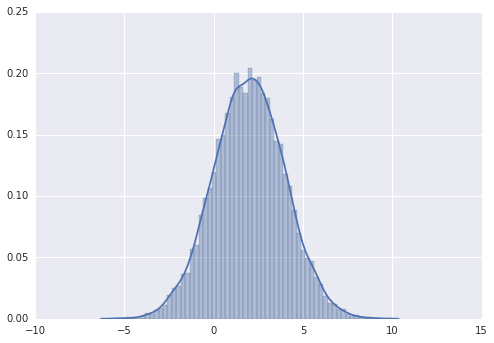In :
sns.set_context('poster')
p = sns.distplot(x, hist=True, rug=False)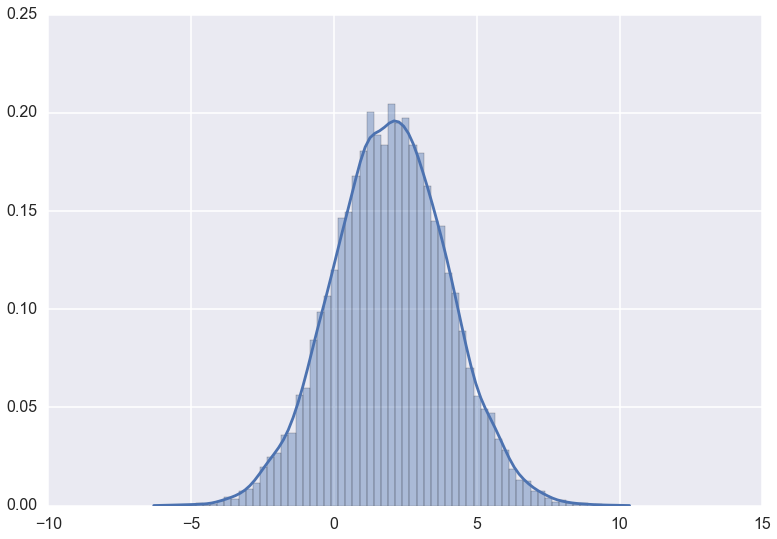In :
sns.set_context('notebook')
p = sns.kdeplot(x)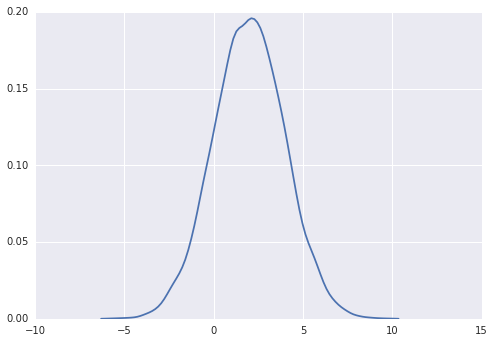In :
p = sns.kdeplot(x, y)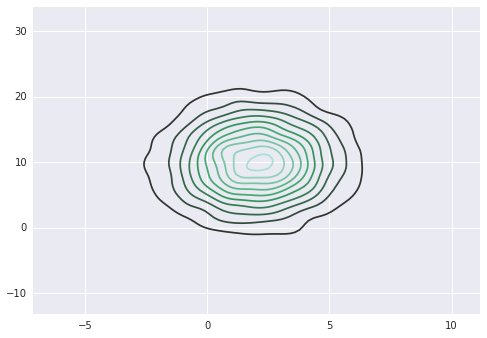### Bokeh¶

In :
from bokeh import mpl
from bokeh.plotting import output_notebook, show

# Just plot seaborn kde
sns.kdeplot(x)

show(mpl.to_bokeh(notebook=True))